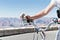## Understanding what really happens in a neural network

This article aims to explain how the activation functions work in a neural network.

If you want to understand the basics of a neural network then please read:

# What Is An Activation Function?

Activation function is nothing but a mathematical function that takes in an input and produces an output. The function is activated when the computed result reaches the specified threshold.

The input in this instance is the weighted sum plus bias:

# Understanding The Formula

As an instance, if the inputs are:

And the weights are:

Then a weighted sum is computed as:

Subsequently, a bias (constant) is added to the weighted sum

Finally, the computed value is fed into the activation function, which then prepares an output.

Think of the activation function as a mathematical operation that normalises the input and produces an output. The output is then passed forward onto the neurons on the subsequent layer.

# What Are Activation Function Thresholds?

The thresholds are pre-defined numerical values in the function. This very nature of the activation functions can add non-linearity to the output. Subsequently, this very feature of activation function makes neural network solve non-linear problems. Non-linear problems are those where there is no direct linear relationship between the input and output.

To handle these complex scenarios, a number of activation functions are introduced which can be configured on the inputs.Photo by Boxed Water Is Better on Unsplash

# Activation Function Types

Let’s review a number of common activation functions. Before I explain each of the activation function, have a look at this table. I am demonstrating how the values differ for the five most well known activation functions which I will be explaining in detail.

Each activation function has its own formula which is used to convert the input.

Let’s understand each of them in detail.

## 1 Linear Activation Function:

The activation function simply scales an input by a factor, implying that there is a linear relationship between the inputs and the output.

This is the mathematical formula:

y is a scalar value, as an instance 2, and x is the input.

This is how the graph looks if y = 2:

## 2 Sigmoid Activation Function:

The sigmoid activation function is “S” shaped. It can add non-linearity to the output and returns a binary value of 0 or 1.

## Consider this non linear example

Let’s assume you buy an European call option. The concept of an European call option is that a premium amount P is paid to buy an option on an underlying, such as on a stock of a company.

The buyer and seller agree on a strike price. Strike price is the amount when the buyer of the option can exercise it.

Now, let’s understand this scenario in practice:

When the price of the underlying stock goes above the strike price, the buyer ends up making profit. However as soon as the price goes below the strike price, the loss is capped and only the premium P is lost. This is a non linear relationship.

This binary relationship of whether to exercise an option or not, can be computed by the sigmoid activation function:

If your output is going to be either 0 or 1 then simply use the sigmoid activation function.

This is the example graph:

## 3 Tanh Activation Function:

Tanh is an extension of the sigmoid activation function. Hence Tanh can be used to add non-linearity to the output. The output is within the range of -1 to 1. Tanh function shifts the result of the sigmoid activation function:

## 4 Rectified Linear Unit Activation Function (RELU)

RELU is one of the most used activation functions. It is preferred to use RELU in the hidden layer. The concept is very straight forward. It also adds non-linearity to the output. However the result can range from 0 to infinity.

If you are unsure of which activation function you want to use then use RELU.

## 5. Softmax Activation Function:

Softmax is an extension of the Sigmoid activation function. Softmax function adds non-linearity to the output, however it is mainly used for classification examples where multiple classes of results can be computed.

Understand with an example

Let’s assume you are building a neural network that is expected to predict the possibility of rainfall in the future. The softmax activation function can be used in the output layer as it can compute the probability of the event occurring in the future.

The activation functions normalise the input and produces a range of values from 0 to 1.

The weights along with the bias can change the way neural networks operate.

If you want to understand what weights and bias are then please read:

If you want to understand what neural network layers are then please read:

If you want to understand how neural network neurons work then please read:

# Summary

This article provided an understanding of how activation functions work in a neural network.

Hope it helps.

Written by

## FinTechExplained

#### This blog aims to bridge the gap between technologists, mathematicians and financial experts and helps them understand how fundamental concepts work within each field. Articles

Welcome to a place where words matter. On Medium, smart voices and original ideas take center stage - with no ads in sight. Watch
Follow all the topics you care about, and we’ll deliver the best stories for you to your homepage and inbox. Explore
Get unlimited access to the best stories on Medium — and support writers while you’re at it. Just \$5/month. Upgrade